Browse by Topic
Related Topics

# On the Importance of Being Edgy—Electrostatic and Magnetostatic Problems with Sharp Edges

(This is the first post in a three-part series about electrostatic and magnetostatic problems involving sharp edges.)

Mathematica can do a lot of different computations. Easy and complicated ones, numeric and symbolic ones, applied and theoretical ones, small and large ones. All by carrying out a Mathematica program.

Wolfram|Alpha too carries out a lot of computations (actually, tens of millions every day), all specified through free-form inputs, not Mathematica programs. Wolfram|Alpha is heavily based on Mathematica, and many of the mathematical calculations that Wolfram|Alpha carries out rely on the mathematical power of Mathematica. And while Wolfram|Alpha can carry out a vast amount of calculations, it cannot carry out all possible calculations, either because it does not (yet) know how to do a calculation or because the (underlying Mathematica) calculation would take a longer time than available through Wolfram|Alpha. So for a detailed investigation of a more complicated engineering, physics, or chemistry problem, having a copy of Mathematica handy is mandatory.

But there is also the reverse relation between Mathematica and Wolfram|Alpha: Wolfram|Alpha’s knowledge, especially its data knowledge, allows it to carry out investigations and calculations that can substantially increase the power of pure Mathematica. And all of this is because Wolfram|Alpha’s knowledge is accessible through the WolframAlpha[] function within Mathematica.

There are many kinds of data accessible from Wolfram|Alpha: values of materials, economic time series, weather data, and so on. All are at your fingertips when they are needed. But there is also a lot of mathematical data, such as properties of curves, surfaces, mathematical functions, and mathematical concepts.

And there is data about physics formulas: ever since its introduction last year, the physical systems data has been quite popular. If you quickly need the equations of motion for the three-body problem, the solution of the equations of motion for a particle in a box, the Hamiltonian of the double pendulum, or propagator of the harmonic oscillator, Wolfram|Alpha has these formulas, in SI units, ready for you.

Obviously, in Mathematica using WolframAlpha[query] you just “see” the Wolfram|Alpha result. For instance, to be reminded about a physics formula, say, the equation of motion for a sinusoidal driven harmonic oscillator, you see the result in the same way as you would on the Wolfram|Alpha website. (Note that all symbols are nicely explained through their physical quantities and dimensions to make the result self-contained and immediately understandable.)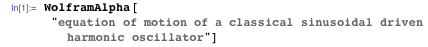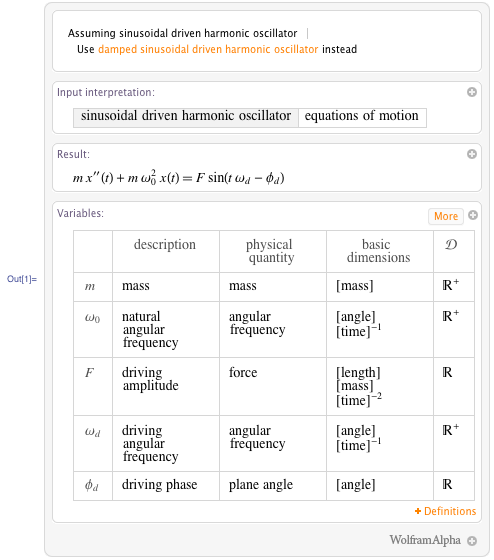But in Mathematica, we can go much further. You can retrieve a Mathematica-program version of each of the last results by using the WolframAlpha[] function with a second argument. (See Lou D’Andria’s talk from the last technology conference on the many facets of WolframAlpha[].) Here we will concentrate on some physics applications. This is the equation of motion of a harmonic oscillator, delivered by Wolfram|Alpha to Mathematica in an immediately computable form.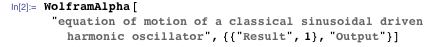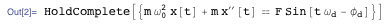You can solve the equation using DSolve: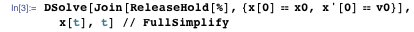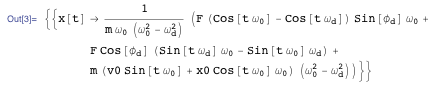You can then easily continue by studying the phase portraits of the oscillator as a function of the initial conditions and the driving force parameters.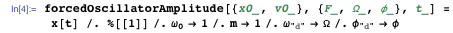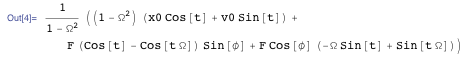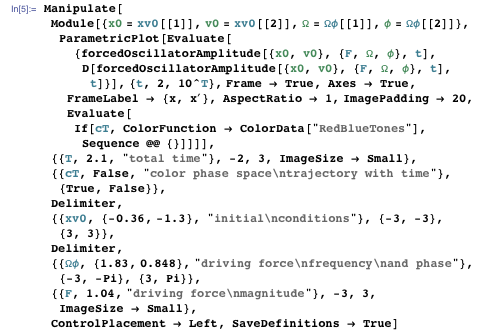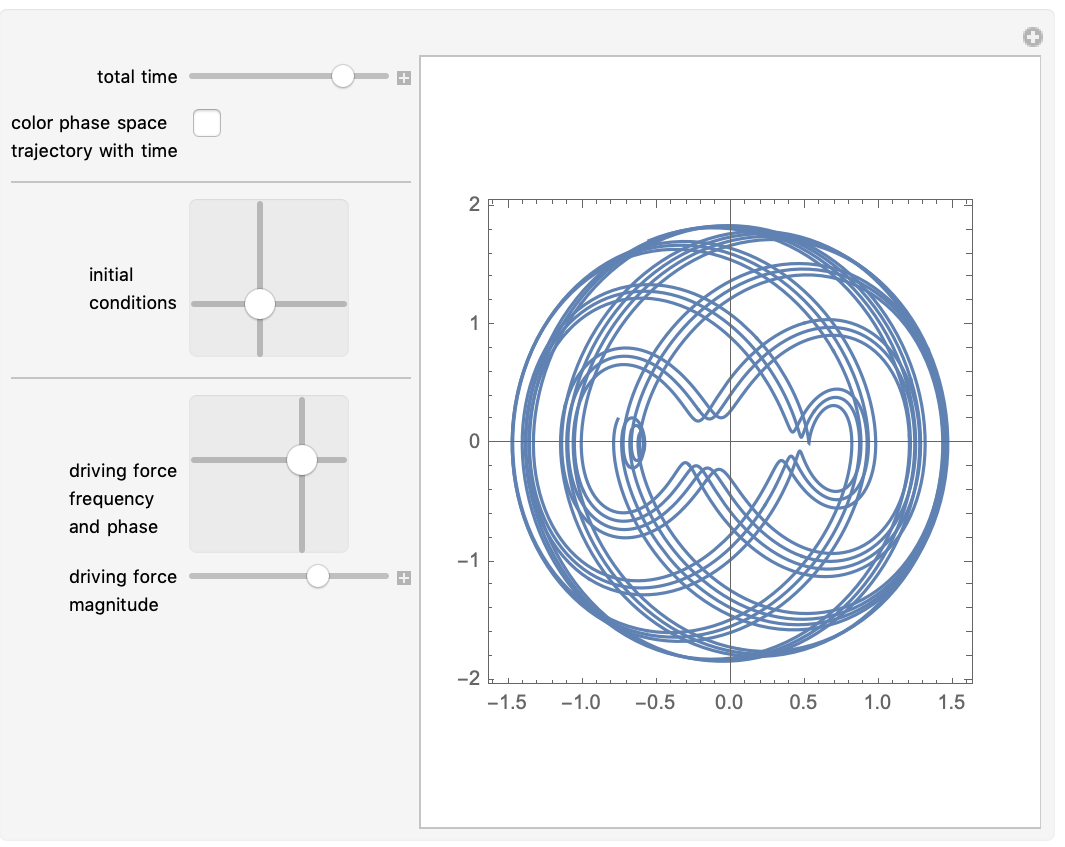While some properties of physical systems are easy to remember and/or easy to derive, some others aren’t so easily remembered (because they are large) or aren’t easy to re-derive (because the derivations can be quite complicated). Think, for instance, about various electrostatic and magnetostatic configurations, which will be the focus of this blog series. The potentials and field strengths of model configurations are often complicated solutions of Poisson’s equation. Even geometrically simple charge and current distributions that are often needed for a variety of physics and engineering problems can result in quite nontrivial mathematical expressions. Needless to say, the results of Wolfram|Alpha are as generic as possible. For instance, the magnetic induction of a Helmholtz coil is not just given along the vertical axis as often seen on various websites (such as Wikipedia), but for every point, even if the formulas get a bit larger and barely fit on a Wolfram|Alpha result page and contain elliptic integrals (we will come back to this example in more detail below). Having complete, correct expressions for the electric and magnetic vector potential and the field strengths at your fingertips available as Mathematica expressions turns out to be extremely useful, as you can then use Mathematica‘s computational capabilities to go on and investigate more properties and effects.

Using Mathematica‘s symbolic and numeric capabilities allows us to investigate a plethora of electromagnetic situations and effects. And while classical electrodynamics is a 100+ year old and well established theory, there are still many applications that are found (like the recent proposal to use evanescent waves to charge a laptop without a cable, or to build invisibility cloaks), new solutions and field configurations (such as knotted beams of light), theoretical derivations to be discovered (such as how retardation can be consistent with the Coulomb gauge), and even fundamental questions to be answered (such as what the momentum of a photon in a dielectric is); all of the last examples just happened in the last few years. So, “playing around” with some electric and magnetic field configurations is still worthwhile.

After some warm-ups, we will justify the title of this blog series and discuss three charge and current distributions that have edges: a rectangular current loop (actually two interlocking copies of it), a charged cube, and a rectangular bar magnet.

Now let’s look at some concrete examples to demonstrate the above points. Here is the electric potential of a homogeneously charged finite line segment.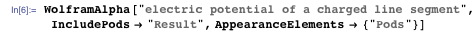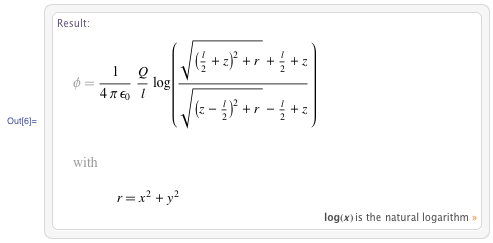And here is the potential as a Mathematica expression: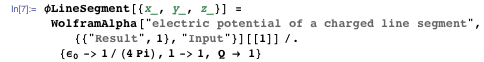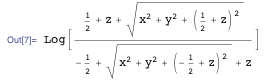For this quite simple example you can directly integrate Poisson’s equation (if you remember the Green’s function 1 / [rr&#8242] for Poisson’s equation) to obtain the potential using Integrate; this will no longer be the case for more complicated charge distributions to be discussed below.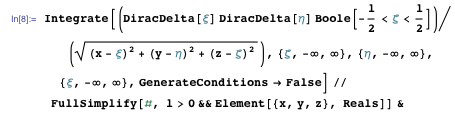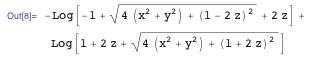Now we can use Mathematica to make a detailed plot of the equipotential curves around the line segment (assuming without loss of generality unit length and charge).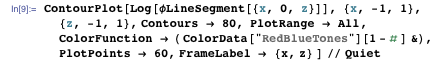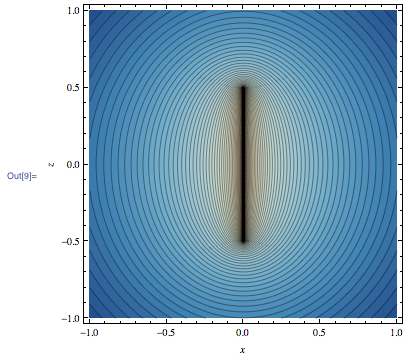This is a 3D plot of the potential over the r, z plane.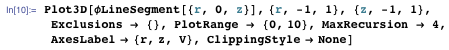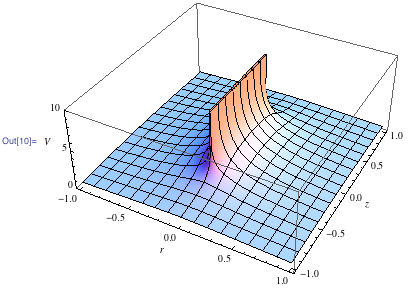What are the orbits of a test charge in the field of such a 1D charged line? Calculating the gradient of the electric field and numerically solving the equations of motion for some random initial conditions and plotting the orbits strongly suggests that the trajectories are generally not periodic.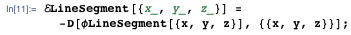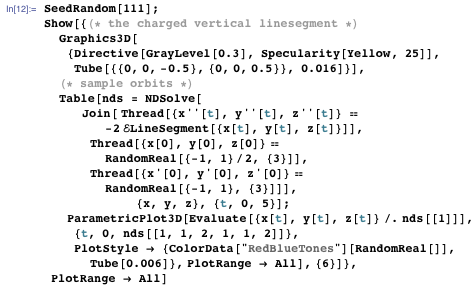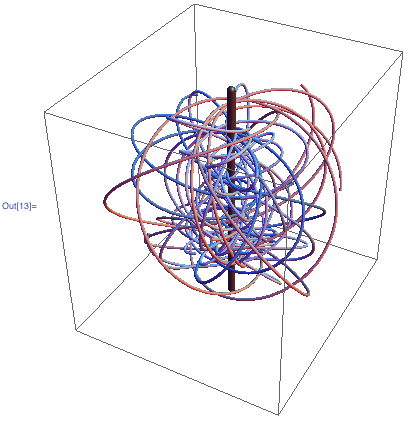By scaling and rotating coordinates, the potential of the above line segment can be used to calculate the potential of any line segment Line[{p1, p2}].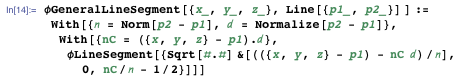Using a few line segments, say the edges of a dodecahedron, we can now easily visualize the equipotential surfaces of a charged wireframe dodecahedron. Here is a sketch of the wireframe of a dodecahedron.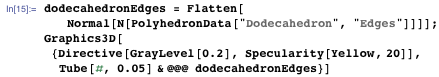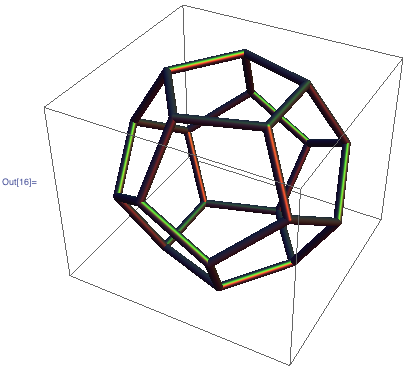And here is an equipotential surface.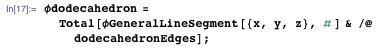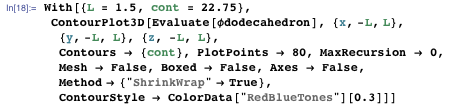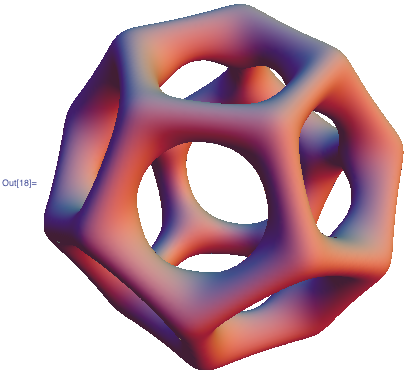We get more “fun-looking” equipotential surfaces if we charge the edges randomly positive or negative, but they are less recognizable as dodecahedrons.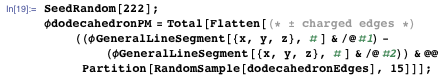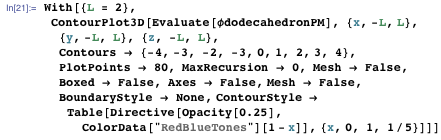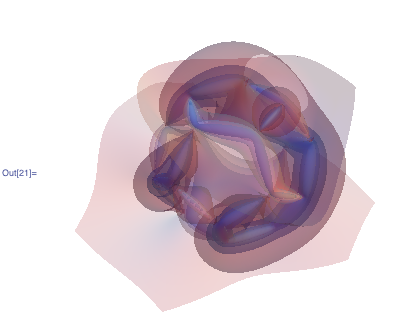The simplest genuine 3D charge configuration (other than a pure point charge) is surely a homogeneously charged ball.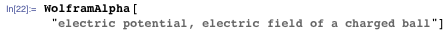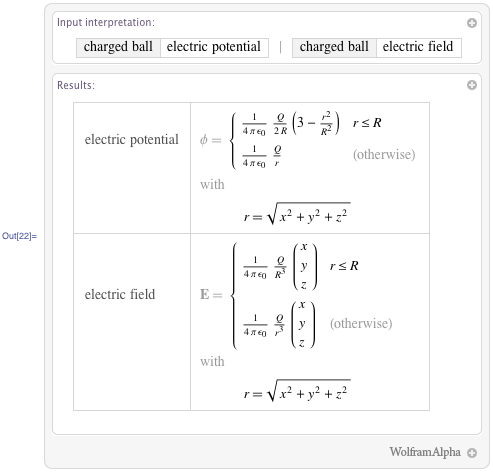The potential inside the ball is quadratic and the potential outside is a 1/r Kepler potential.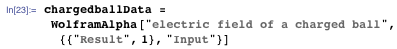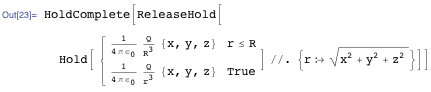By Bertrand’s theorem, these are exactly the two central potentials in 3D that generically allow for closed orbits independent of the initial conditions. Assuming a test charge can enter the charged ball without any further mechanical force, what do the orbits now look like? Are they still closed orbits? The following Manipulate allows us to play with orbits in a plane through the origin of the charged sphere.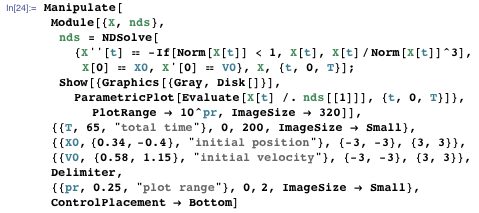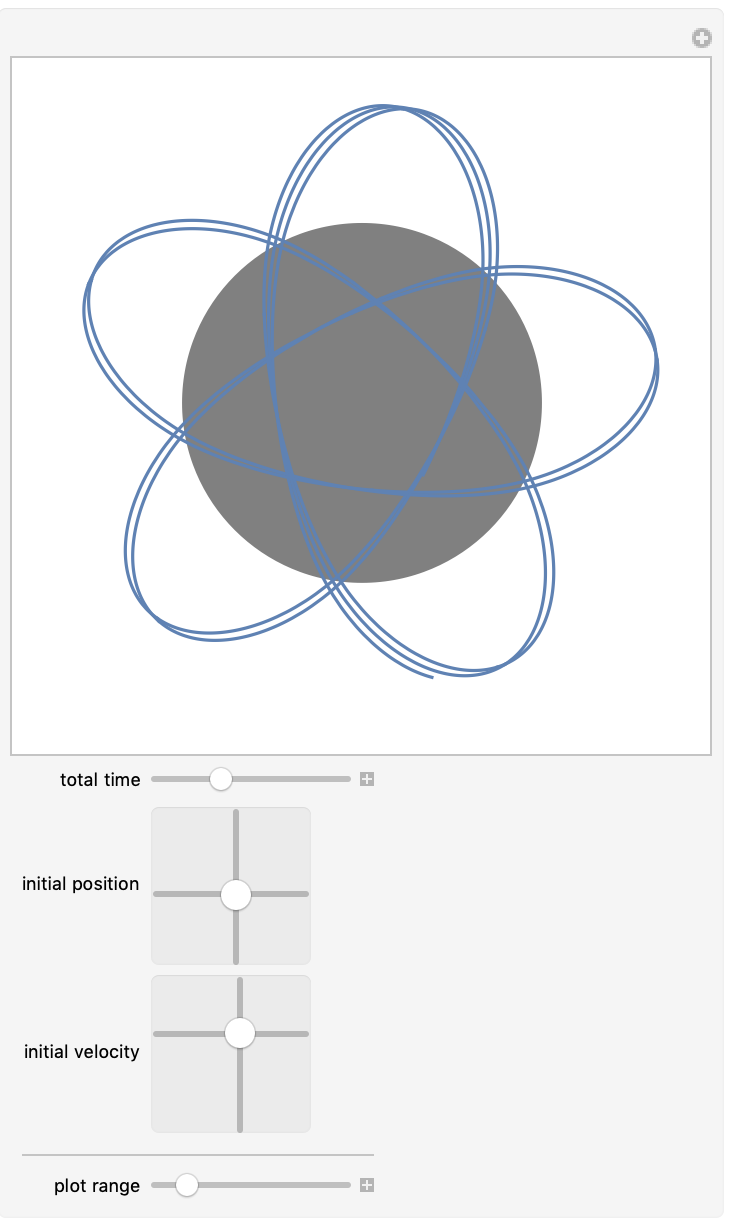A homogeneously charged disk seems like an even simpler problem than a homogeneously charged sphere. But this is not the case; the closed form for the potential contains various elliptic integrals. Here is the electric potential of a charged disk as first derived by Lass and Blitzer in 1983. Again, we consider a homogeneously charged body, meaning the charges are fixed in space and cannot move.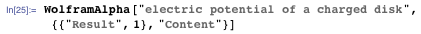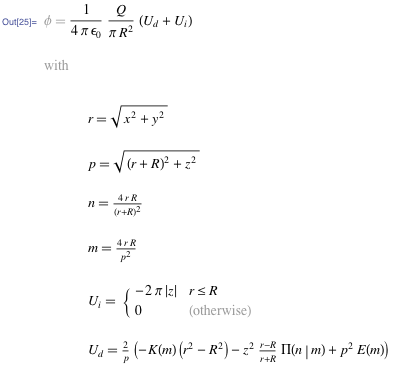We retrieve the Mathematica form; note that the returned Mathematica expression is in held form to represent a concise form that uses natural intermediate variables.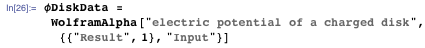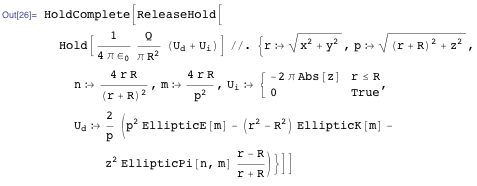We use unit radius and charge and set the electric constant to 4π for convenience.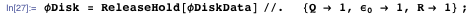And here is a cross-section plot of the equipotential curves.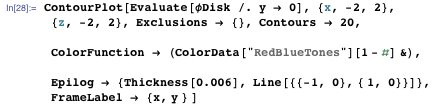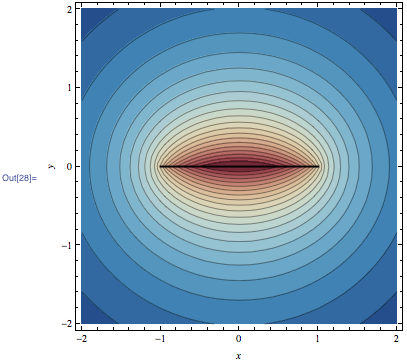We simplify the expression for the field strength and express it in cylindrical coordinates.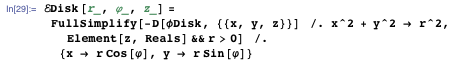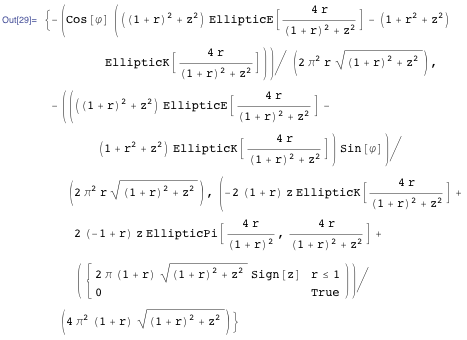We plot the curves of constant magnitude of the field strengths, which look quite different from the curves of equal potential. Within Mathematica, we can now also easily make a 3D plot of the surfaces of constant magnitude of the electric field using ContourPlot3D.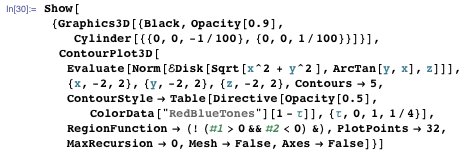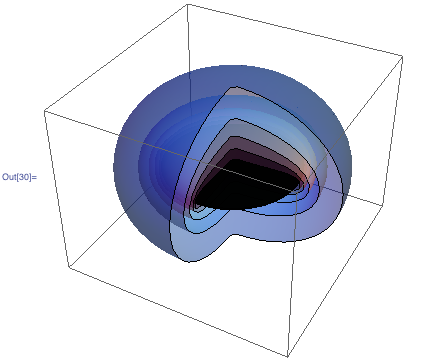One of the simplest electromagnetic systems is a single moving-point charge. Yet at the same time it represents one of the most important and fascinating electromagnetic systems. The resulting potentials and field strengths are the famous Liénard–Wiechert potentials.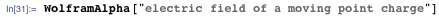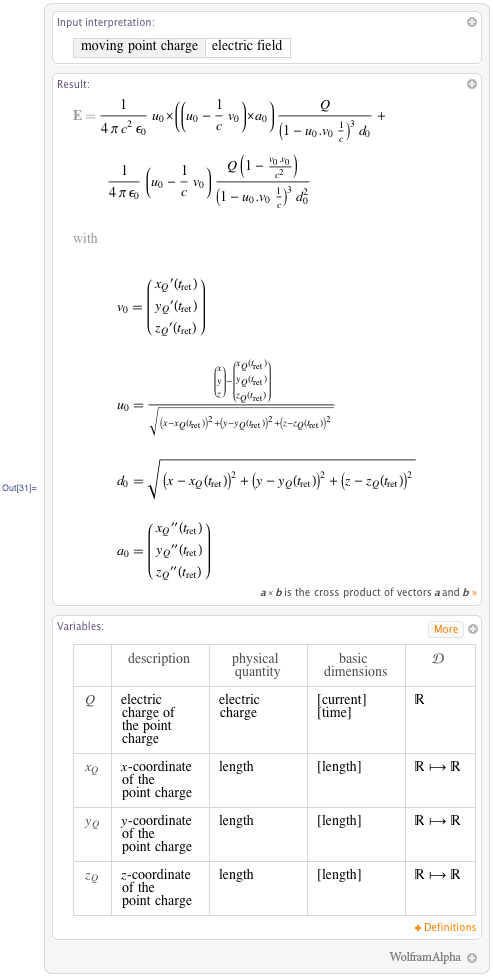The Mathematica form is even more compact than the nicely formatted version.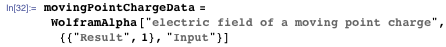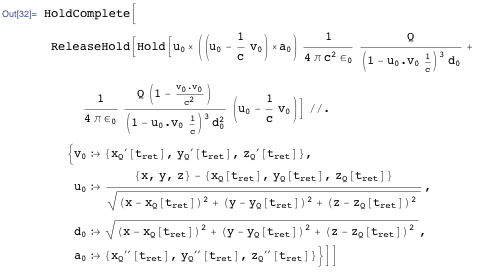An accelerating charge radiates electromagnetic radiation, and the detailed time-dependence of the fields allows us to watch its birth in time-lapse. For a simple example, we will move a point charge in unit time along the x axis. Before t = 0 and after t = 1 the charge is at rest. We will use a sufficiently smooth x(t) dependence so that x(t) is still smooth. Here are plots of the position and velocity (velocity measured in multiples of the speed of light).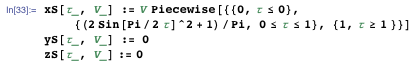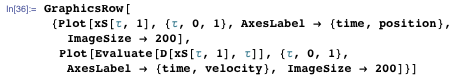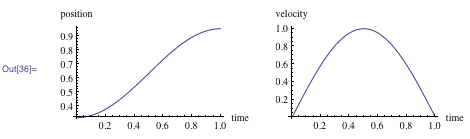For the above Liénard—Wiechert formulas, we need the retarded time. Using a bisection method, we can quickly and reliably numerically determine the retarded times.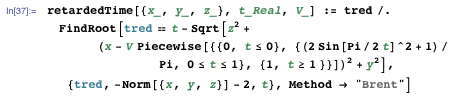For the next graphic, we will visualize the electric field of the moving-point charge.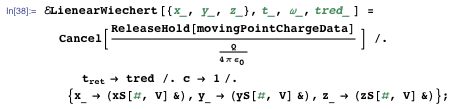For a faster graphics calculation, we also define a compiled version.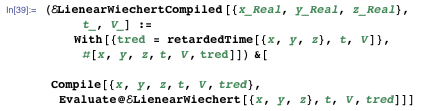Because of the symmetry of our moving charge, we plot the electric field in a plane containing the line of motion of the charge. Here is a plot of the electric field direction of a charge with maximal speed v = 0.9c after the charge rests again (position of the point charge is indicated as a black dot).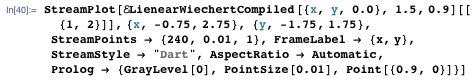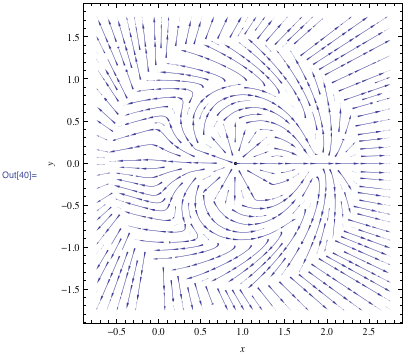Watching the magnitude of the electric field (in the lab frame) at various times nicely shows how the radiated-away electromagnetic wave forms. The white point near the center of the following graphics indicates the current position of the point charge. For better visibility of the radiated-away wave, we scale the field strength nonlinearly for coloring purposes.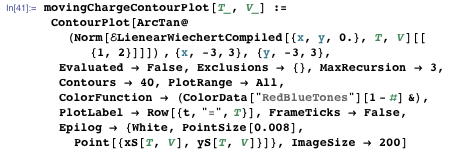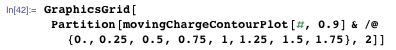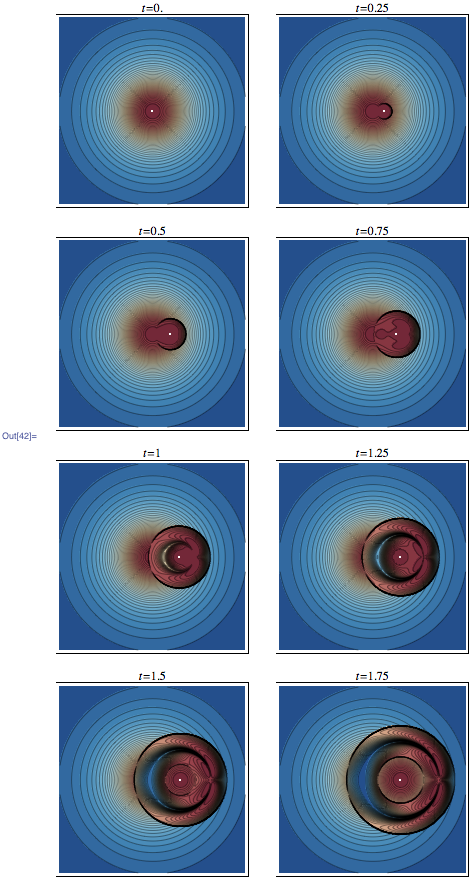For times larger than 1, you can see the wavefronts that we expect from a wave equation solution in odd space dimensions, meaning the inside of the ring is again the static electric field of the charge at rest after its movement.

Now let’s look at a magnetic field example. The magnetic field of a Helmholtz coil is a relatively complicated function, so we display only part of the result. While many physics textbooks and Wikipedia just give the result for the field along the symmetry axis, the Wolfram|Alpha result is valid everywhere, and the result again contains elliptic functions (as a rule of thumb, the closed forms of the fields of most circular charges and currents typically contain elliptic integrals.)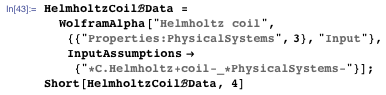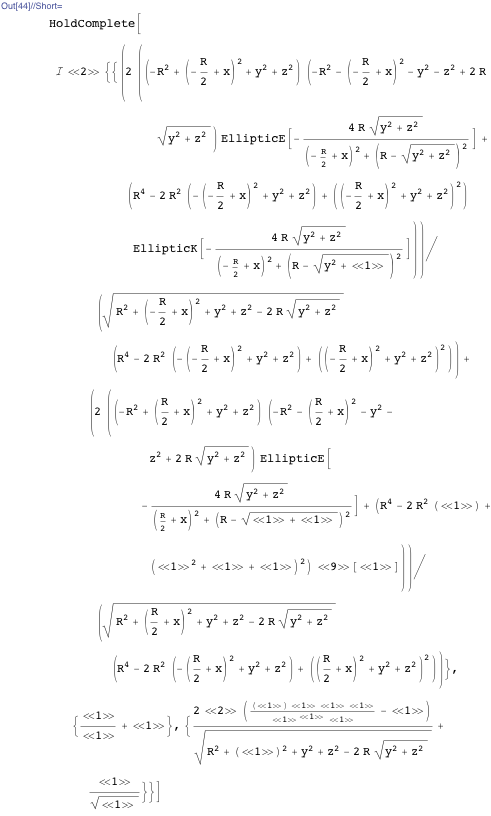Here is a sketch of the magnetic field lines in a plane containing the symmetry axis.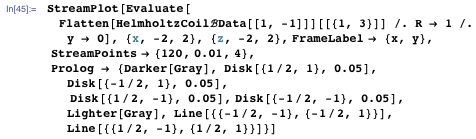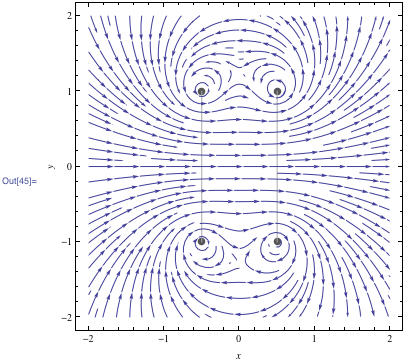The most important feature of a Helmholtz coil is the high degree of constancy in the center. The following contour plots show this nicely. Note also the two small circular minima of |B|.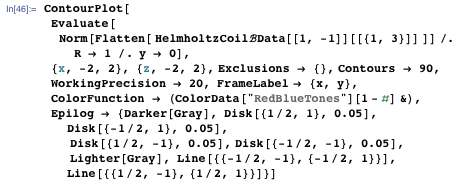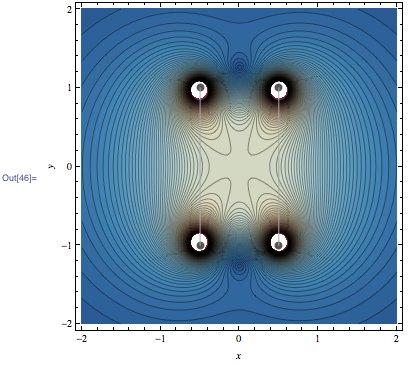Here is the 3D analog of the last graphic—the surfaces of constant |B|. (For better visibility, we cut out parts of the surfaces.)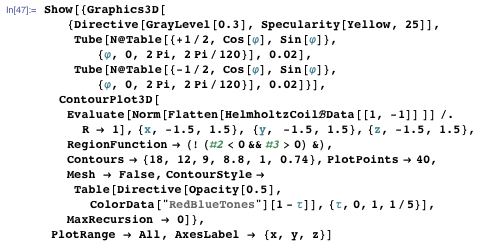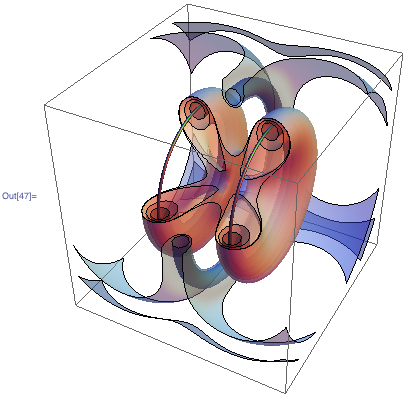Expanding the magnetic field at the center shows the absence of second-order terms that explain the high degree of uniformity.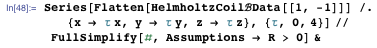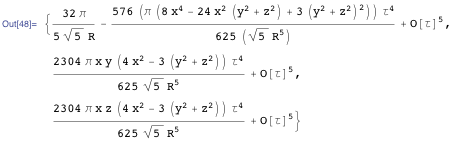The last examples of fields were relatively complicated and contained elliptic functions. To justify the title, let us now look at the simplest current configuration with an edge, a rectangular current loop.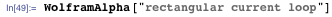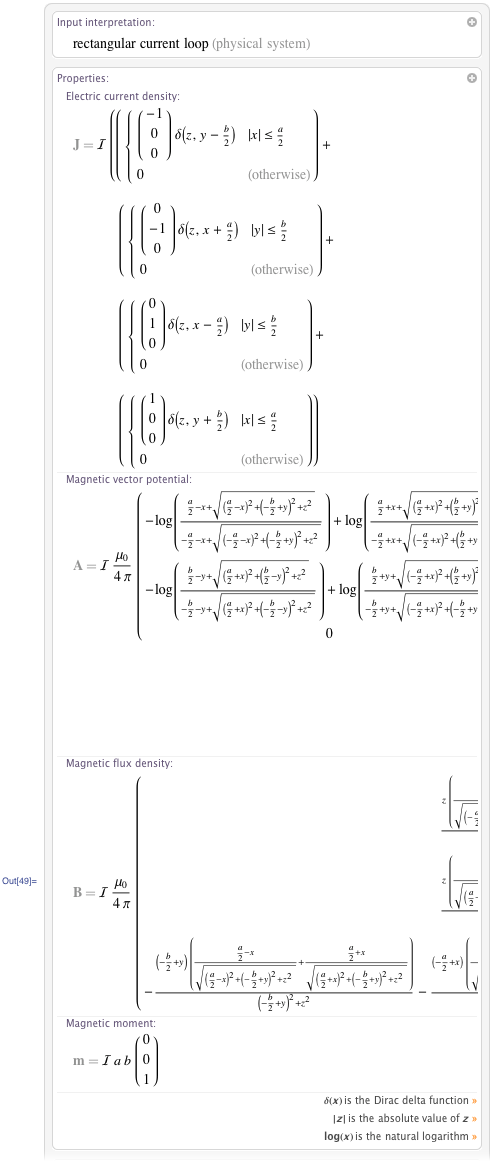The magnetic flux again turns out to be a relatively large expression, but this time it does not contain any higher special functions; it is just a sum of elementary functions, mostly logarithms.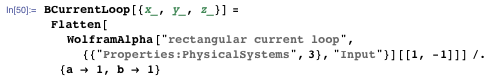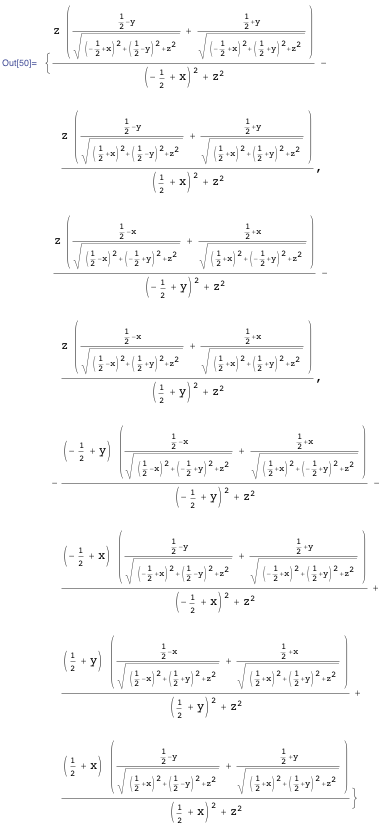A 3D plot of the vector field of the magnetic induction shows locally the influence of the four straight wire pieces.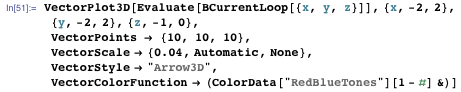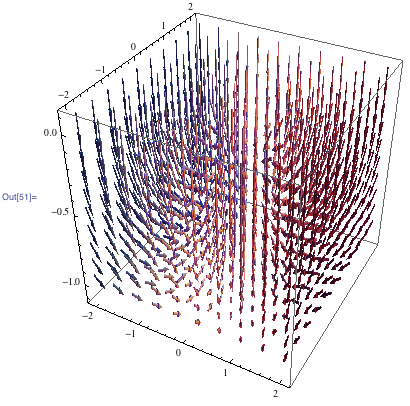For a more interesting configuration, we add a rotated and translated copy of the original current loop.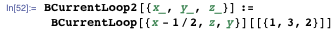Here is a plot of the magnitude of the resulting magnetic induction. (Again we remove parts of the surface to better see the inner surfaces.)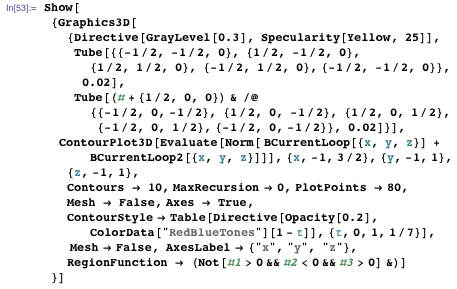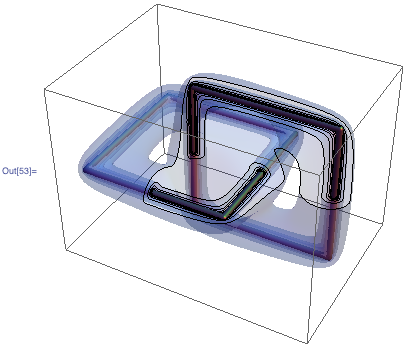While these two interlocking current loops are a simple current distribution, they show some interesting effects. Let’s have a look at the field lines of the magnetic induction of these two current loops. In the literature one often finds statements such as, “A magnetic field has no sources or sinks (Gauss’s law for magnetism), so its field lines have no start or end: they can only form closed loops, or extend to infinity in both directions (quoted from the Wikipedia page for field lines).” This is not really the case. As already pointed out by Slepian in 1951, often magnetic field lines are neither closed nor extend to infinity. Mathematically, the system of equations that describes magnetic field lines is Hamiltonian. It is well known that Hamiltonian systems can exhibit chaotic behavior. So, it follows that the magnetic field can also show chaotic behavior. (The Maxwell equation div B = 0 contains a differential operator and so does not make any statements about individual field lines.)

We program an interactive application that accepts the starting point of a field line and then visualizes the field line. We use a unit current in the first current loop and a current of strength α in the second current loop. When there is no current in the second loop, the field lines are closed (as they are for any planar current). With a non-vanishing current in the second loop, the field lines are no longer closed, but in addition to looping around the first loop, slowly creep along the loop. With further increasing current in the second loop, more complicated field line shapes arise. The small red sphere and the green arrow indicate the initial position and direction of the field line.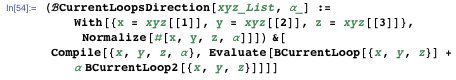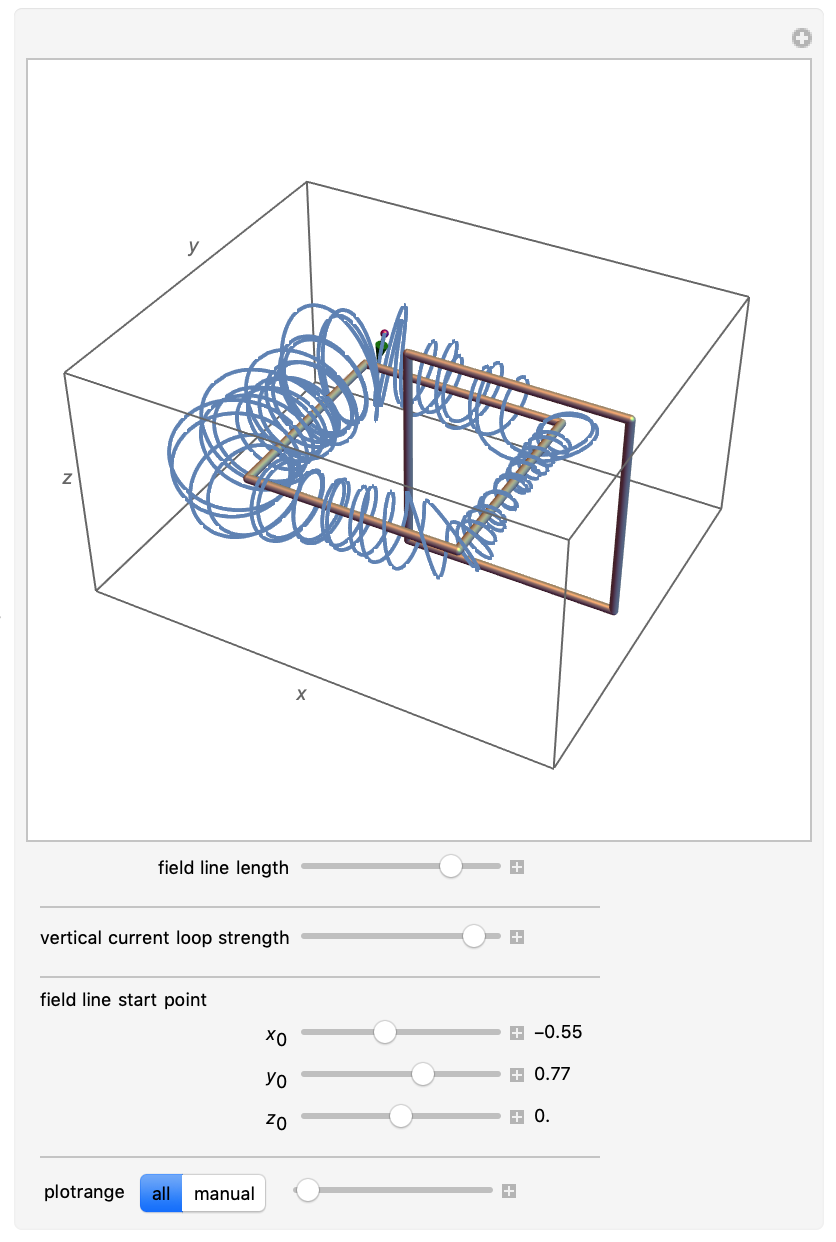This ends today’s excursion into the use of Wolfram|Alpha’s physics formula in Mathematica. In the next blog post, we will look in detail at the potential, the electric field, and the orbits of a homogeneously charged cube.